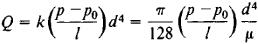# Poiseuille's Law

Also found in: Dictionary, Thesaurus, Medical, Legal, Wikipedia.
Related to Poiseuille's Law: Poiseuille's equation

## Poiseuille's law

[pwä′zə·ēz ‚lȯ]
(fluid mechanics)
The law that the volume flow of an incompressible fluid through a circular tube is equal to π/8 times the pressure differences between the ends of the tube, times the fourth power of the tube's radius divided by the product of the tube's length and the dynamic viscosity of the fluid.

## Poiseuille’s Law

the law governing the flow of a liquid through a thin cylindrical tube. It states that the volume Q per second of a liquid flowing through a cross section of the tube is directly proportional to the pressure difference between p and p0 at the entrance of the tube and at the outlet and to the fourth power of the diameter d of the tube and inversely proportional to the length l of the tube and the viscosity μ of the liquid:This formula was derived by J. L. M. Poiseuille, and the relation of the coefficient k to the viscosity μ was established later by G. Stokes: k = π/(128μ).

Poiseuille’s law is applicable only to a liquid in laminar flow (in practice, for very thin tubes) and on the condition that the length of the tube greatly exceed the length of the initial section, in which the laminar flow develops in the tube. Poiseuille’s law is used in determining the viscosity of liquids at different temperatures by means of capillary viscometers.

Mentioned in ?
References in periodicals archive ?
The observed unidirectional blood flow can be explained for both in vivo and postmortem scenarios upon a number of logical assumptions in order to fit Poiseuille's law: (1) the vessel length (L) and the viscosity ([eta]) of the vessel content in Equation (1) are the same for the HA and PV; (2) the tiny PVP path between the HA and PV is always open and could be bidirectional; (3) empirically the radius of PV is about 10 times larger than that of the HA (e.g., 1mm versus 0.1mm); and (4) the elasticity of the wall can be considered similar in the HA and PV.
Validity of Poiseuille's law for the plants has been thoroughly verified and the hydraulic resistivity of real conducting pathways was found to be bigger than the theoretical values, namely Z = aZP, where a = 2.04 for lianas .
The system produces an absolute leak number based on the application of Poiseuille's law. The impact to packagers is that they will now be able to measure in one quick analysis, two critical variables which determine package performance.
According to Poiseuille's law, increased blood viscosity should result in reduced microvascular flow, noted Dr.

Site: Follow: Share:
Open / Close